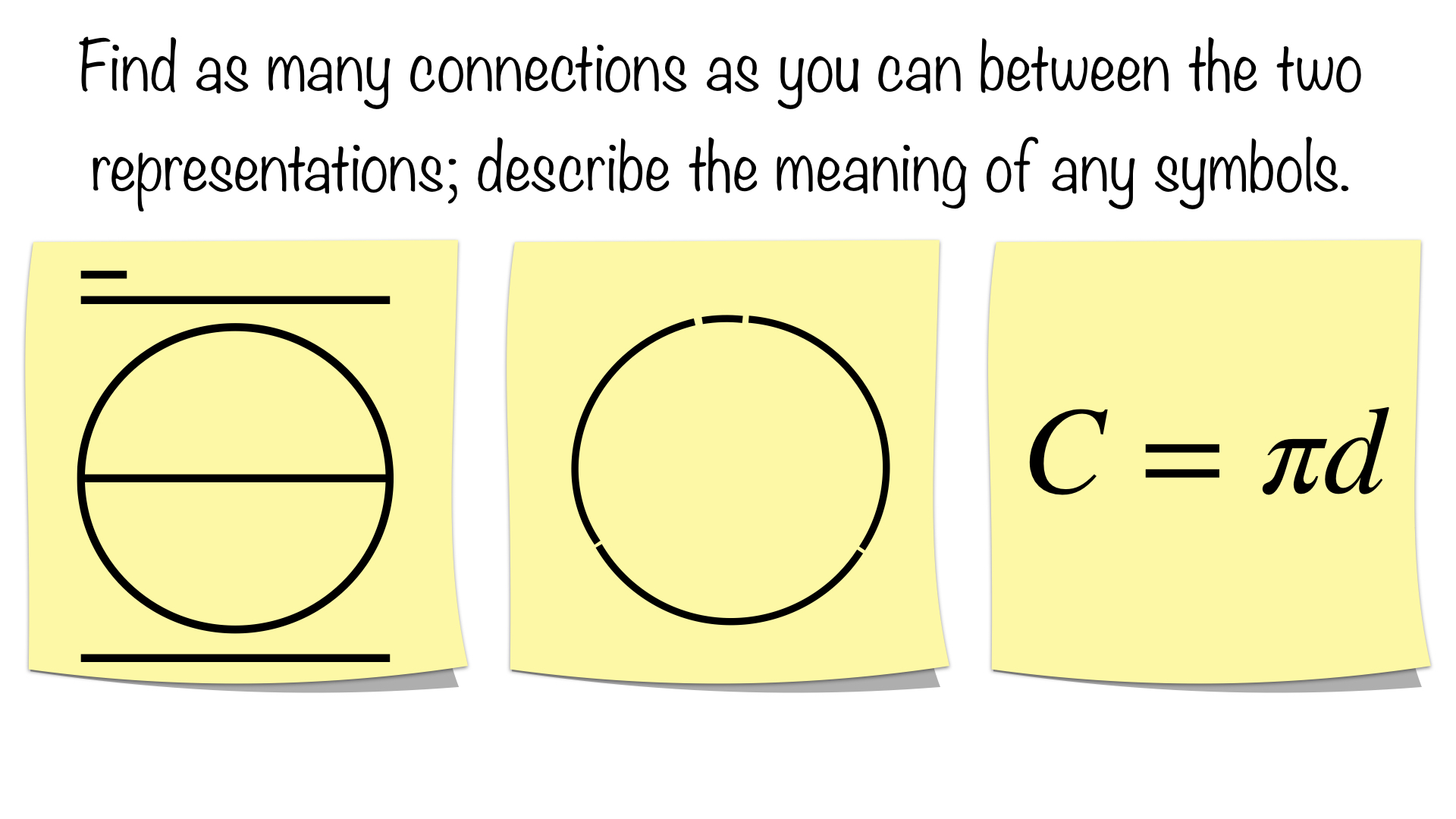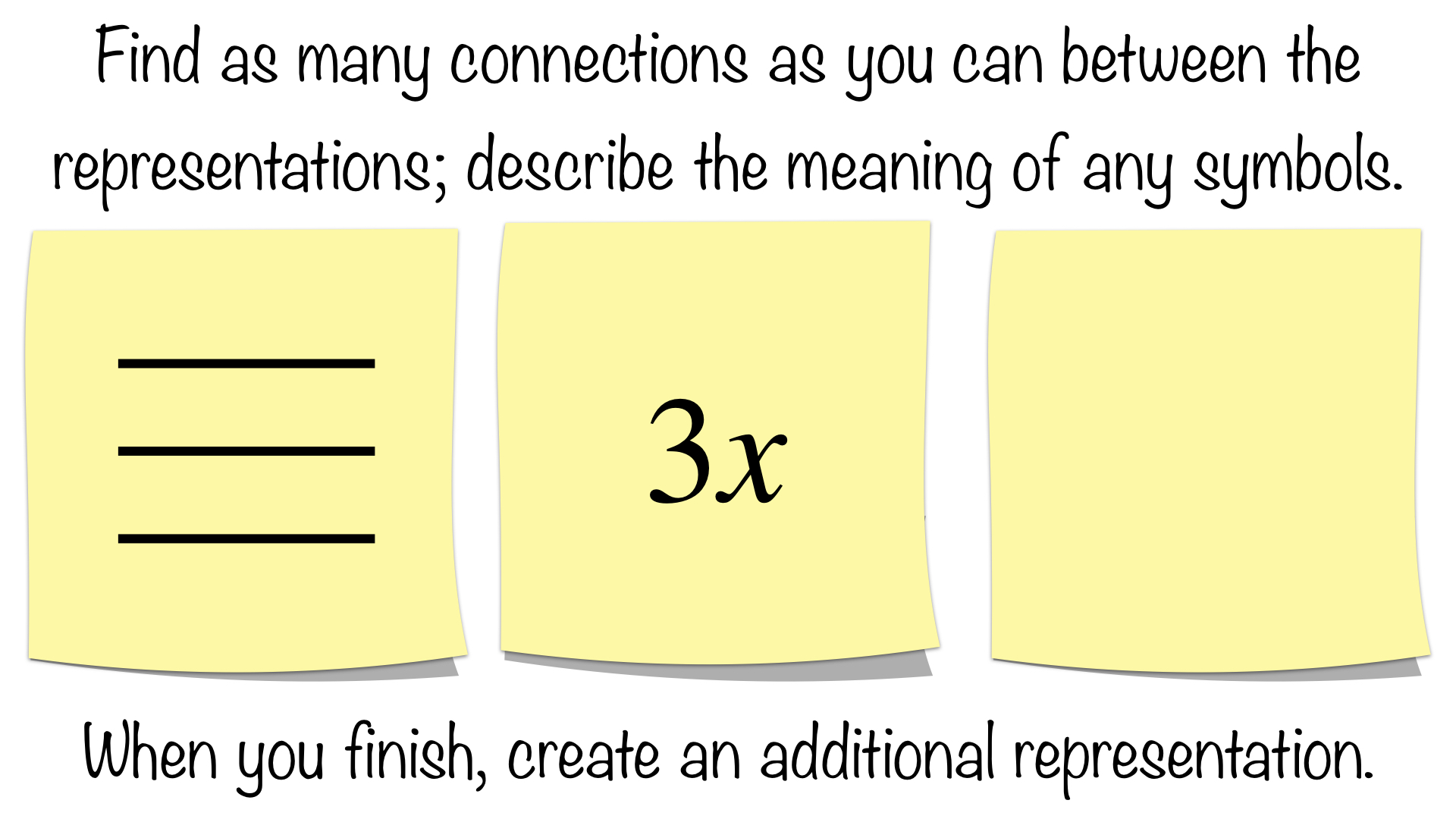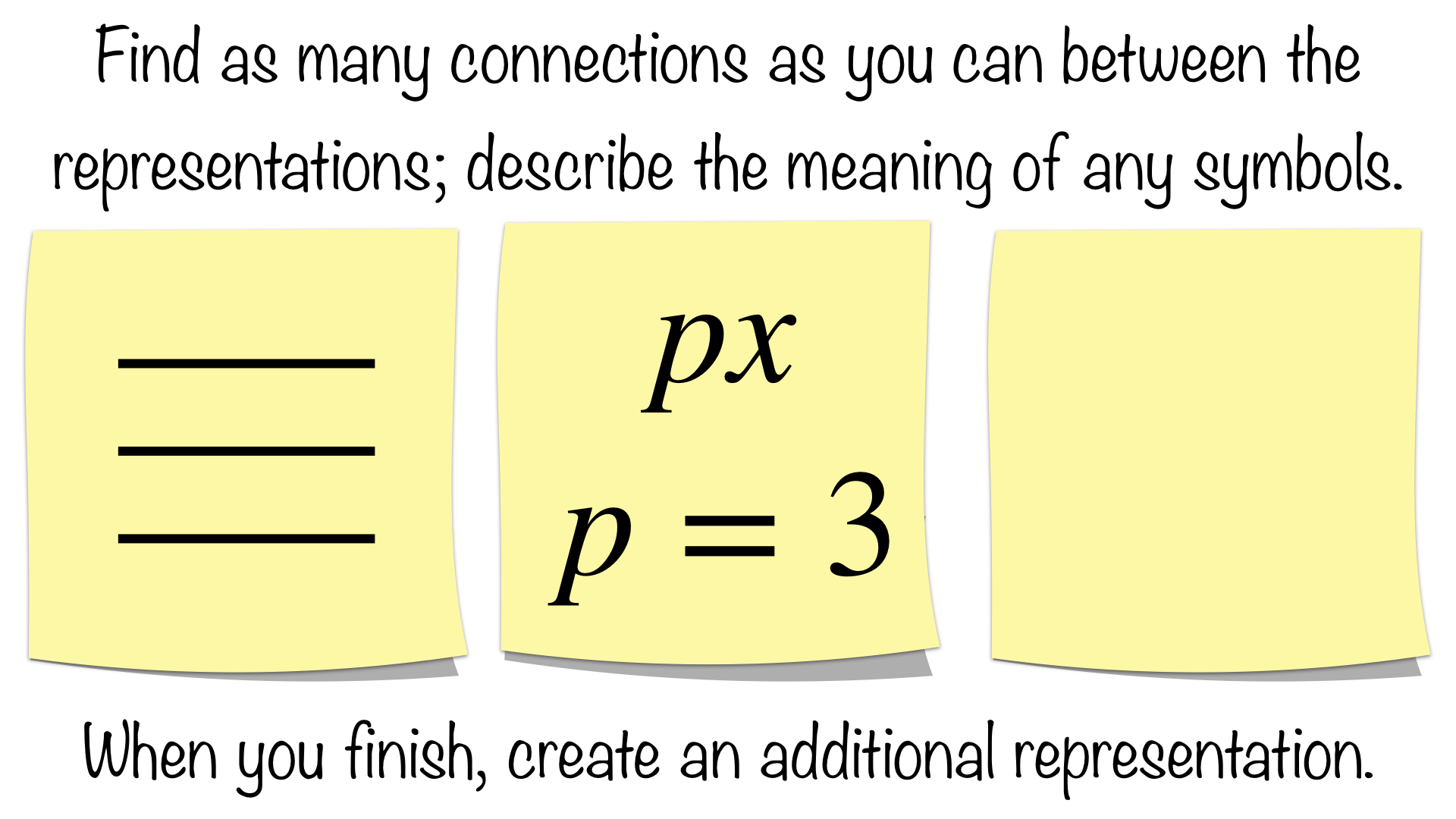Select Page

Common Core Standard: 7.G.B.4

These Sticky Math activities can each stand alone; however, each of these blogposts suggests how you might put them together to form a lesson.  You can always adapt them to suit your needs.

This Sticky Math Lesson helps build fluency from conceptual understanding as students make connections between representations of circumference. Remembering a relationship is easier than remembering an abstract formula.  Apply and extend understanding of a linear term in algebra as students deal with abstraction to a coefficient of pi.

This Sticky Math Lesson examines the circumference of a circle conceptually by exploring the meaning of pi. This lesson can introduce vocabulary through the drawings, offers an opportunity for students to use precise vocabulary (MP6), and acts as a formative assessment of academic vocabulary.

UNDERSTANDING THE PICTURE

Our first Sticky Math Pair might seem confusing at first at their seems to be 2 longer lines and one smaller line outside of a circle, which is true; however, the aha comes by realizing these are three diameters plus a little more:Connections could include:

• On the left sticky, the two lines outside the circle are congruent to the diameter
• On the left sticky there are three diameters plus a little bit more
• There is a circle and a diameter in the the left sticky
• If you “curved” the four lines in the left sticky, then they would be the arcs of the circle in the right sticky
• Three diameters plus a little bit more are equivalent to the circumference of the circle

Students could create their own representations; these might include:

• Describing the relationship in words
• Describing the relationship with symbols

The big takeaway here is that three diameters plus a little bit more are equivalent to the circumference of the circle, which prepares them to define pi.

DEFINING PI

This Sticky Math Pair defines pi as the ratio of circumference to diameter.  In this case, the ratio is a fraction too.  The challenge might be in “seeing” the division of circumference by diameter:Connections could include:

• The C in the left sticky represents the circumference
• The d in the left sticky represents the diameter
• The pi in the left sticky represents the number of diameters in the right sticky

Students could create their own representations; these might include:

• defining diameter as the ratio of circumference to pi
• defining circumference as the diameter pi times, pi times the diameter, or pi groups of the diameter

Like previous posts, students may not come up with the last two bullets; however, you can introduce them one at a time if you like on individual stickies.  Note the extra wording in the final bullet above.  We might be quick to emphasize pi times the diameter asking students to just memorize it; however, students should understand it is the diameter pi times or pi groups of the diameter, which is done explicitly in the following stickies.

THE FORMULA FOR CIRCUMFERENCE IN TERMS OF DIAMETER &                                                        UNDERSTANDING A LINEAR TERM

If you have chosen to define pi before now like done above, then this Sticky Math Triad should be an easier task; however, it is still a necessary task in making connections to the symbolic representation of circumference.Connections could include:

• On the left sticky, the two lines outside the circle are congruent to the diameter
• On the left sticky there are three diameters plus a little bit more
• There is a circle and a diameter in the the left sticky
• If you “curved” the four lines in the left sticky, then they would be the arcs of the circle in the right sticky
• Three diameters plus a little bit more are equivalent to the circumference of the circle
• the C in the right sticky represents the circumference
• the d in the right sticky represents the diameter
• the pi in the right sticky represents the number of diameters in the middle sticky

The key here is to notice that the circumference is equivalent to the diameter pi times or pi groups of the diameter.  A related, supporting task for understanding the meaning of πmight be the Sticky Math Pairs that follow:The idea here is to realize one possible meaning of the coefficient; in this case, 3 means the number of lines with an unknown length of x.

We abstract this a bit more in the next Sticky Math Pair:This takes us back to our original & final level of abstraction of thinking of a symbol as a coefficient or number of groups.So πd, means about 3.14 groups of diameter to get around the circumference of a circle.  We will see this need/opportunity for understanding algebraic terms again when we visit relating the area of a circle to the area of a rectangle in my next blogpost.  In preparation for that work, we consider circumference in terms of radius.

You may want to declare success in getting students to truly understand the formula for circumference in terms of diameter; however, this Sticky Math Triad supports fluency as students define circumference in terms of something new, and it prepares them for understanding the area of a circle (see my next blogpost).  The first sticky in this Sticky Math Triad should be familiar by now:Connections could include:

• on the left sticky, there are three diameters plus a little bit more make up the circumference
• there is a circle in the the left sticky (low floor here)
• six radii plus a little bit more are equivalent to the circumference of the circle
• the C in the middle sticky represents the circumference
• the d in the middle sticky represents the diameter
• the pi in the middle sticky represents the number of diameters in the left sticky
• the r in the middle sticky represents the radius on the right sticky
• the diameters have been replaced by 2 radii in the middle & right stickies

I am convinced students struggle with understanding substitution.  I know many can substitute a value into an expression; however, I am not sure how many truly understand what they are doing.  This becomes especially clear once you reach solving linear systems via substitution or things like the Transitive Property.  What is shown in the Sticky Math Triad above is a visual example to support understanding the substitution being done, replacing the diameter with two radii.

Incidentally, here is a great strategy to boost the understanding of changing the form of an equation or formula – challenge yourself to do so visually as well as symbolically.  I give an example of this in my next blogpost as we relate the area of a circle to the area of a rectangle.

Common Core Standards: 7.G.B.4

What connections are your students making?  What modifications are you making to use this with students?  We would love to hear your feedback; please submit a comment below or consider submitting your own Sticky Math activity here.